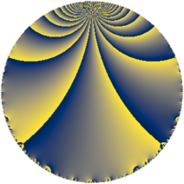# Properties

 Label 143.4.hLevel $143$ Weight $4$ Character orbit 143.h Rep. character $\chi_{143}(14,\cdot)$ Character field $\Q(\zeta_{5})$ Dimension $144$ Newform subspaces $2$ Sturm bound $56$ Trace bound $1$

# Related objects

## Defining parameters

 Level: $$N$$ $$=$$ $$143 = 11 \cdot 13$$ Weight: $$k$$ $$=$$ $$4$$ Character orbit: $$[\chi]$$ $$=$$ 143.h (of order $$5$$ and degree $$4$$) Character conductor: $$\operatorname{cond}(\chi)$$ $$=$$ $$11$$ Character field: $$\Q(\zeta_{5})$$ Newform subspaces: $$2$$ Sturm bound: $$56$$ Trace bound: $$1$$ Distinguishing $$T_p$$: $$2$$

## Dimensions

The following table gives the dimensions of various subspaces of $$M_{4}(143, [\chi])$$.

Total New Old
Modular forms 176 144 32
Cusp forms 160 144 16
Eisenstein series 16 0 16

## Trace form

 $$144 q + 8 q^{2} - 164 q^{4} + 8 q^{5} + 84 q^{6} + 64 q^{7} - 68 q^{8} - 296 q^{9} + O(q^{10})$$ $$144 q + 8 q^{2} - 164 q^{4} + 8 q^{5} + 84 q^{6} + 64 q^{7} - 68 q^{8} - 296 q^{9} + 24 q^{10} - 98 q^{11} - 280 q^{12} + 26 q^{13} + 250 q^{14} + 176 q^{15} + 72 q^{16} - 42 q^{17} + 114 q^{18} - 118 q^{19} + 128 q^{20} - 432 q^{21} - 272 q^{22} + 416 q^{23} + 1008 q^{24} - 692 q^{25} - 354 q^{27} + 294 q^{28} + 610 q^{29} + 516 q^{30} + 504 q^{31} - 656 q^{32} + 1978 q^{33} - 1320 q^{34} + 352 q^{35} - 3198 q^{36} - 504 q^{37} + 654 q^{38} + 312 q^{39} - 510 q^{40} + 1516 q^{41} - 48 q^{42} - 268 q^{43} + 2410 q^{44} + 224 q^{45} - 3550 q^{46} - 392 q^{47} - 1624 q^{48} - 3664 q^{49} + 990 q^{50} + 602 q^{51} + 1716 q^{52} + 2168 q^{53} - 284 q^{54} + 1632 q^{55} + 3188 q^{56} + 666 q^{57} - 3350 q^{58} - 258 q^{59} - 2584 q^{60} + 156 q^{61} - 4754 q^{62} + 5332 q^{63} - 2132 q^{64} - 2080 q^{65} + 4998 q^{66} + 3452 q^{67} + 2336 q^{68} - 2508 q^{69} - 148 q^{70} + 2376 q^{71} + 892 q^{72} + 5552 q^{73} - 504 q^{74} - 542 q^{75} - 2624 q^{76} + 2846 q^{77} + 3640 q^{78} - 3168 q^{79} - 8138 q^{80} - 10182 q^{81} + 7668 q^{82} + 1366 q^{83} - 5212 q^{84} - 3984 q^{85} - 1436 q^{86} + 3736 q^{87} - 4008 q^{88} + 188 q^{89} + 4216 q^{90} - 624 q^{91} + 714 q^{92} - 1044 q^{93} + 5482 q^{94} + 116 q^{95} + 9736 q^{96} + 1986 q^{97} - 7060 q^{98} - 3606 q^{99} + O(q^{100})$$

## Decomposition of $$S_{4}^{\mathrm{new}}(143, [\chi])$$ into newform subspaces

Label Dim $A$ Field CM Traces $q$-expansion
$a_{2}$ $a_{3}$ $a_{5}$ $a_{7}$
143.4.h.a $68$ $8.437$ None $$4$$ $$12$$ $$24$$ $$8$$
143.4.h.b $76$ $8.437$ None $$4$$ $$-12$$ $$-16$$ $$56$$

## Decomposition of $$S_{4}^{\mathrm{old}}(143, [\chi])$$ into lower level spaces

$$S_{4}^{\mathrm{old}}(143, [\chi]) \cong$$ $$S_{4}^{\mathrm{new}}(11, [\chi])$$$$^{\oplus 2}$$